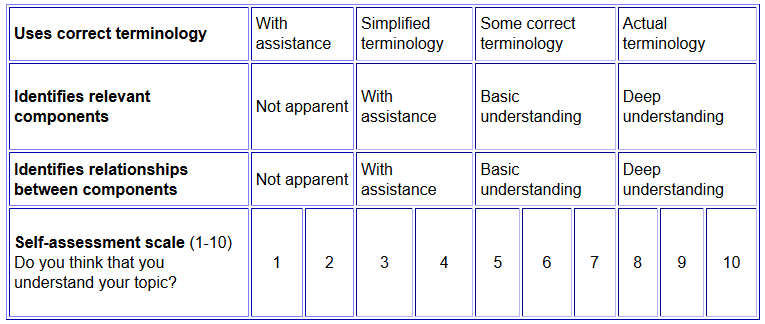# SILO 1.2 (DRAFT)

## Year 1, Term 2: Shapes and objects

### Learning intention: Students recognise, represent and classify familiar two-dimensional shapes and three-dimensional objects.

NSW Syllabus
Australian Curriculum (version 9.0)
"A student recognises, describes and represents shapes including quadrilaterals and other common polygons." (MA1-2DS-01)
"A student recognises, describes and represents familiar three-dimensional objects." (MA1-3DS-01)
"Students learn to make, compare and classify familiar shapes; recognise familiar shapes and objects in the environment, identifying the similarities and differences between them." (AC9M1SP01)

## Week 1

Introduction to topic

Tangram activity

An introductory geometry activity which also involved careful listening and following instructions is as follows:

• Give each student a piece of paper and ask them to rule up their own Tangrams sheet by following verbal instructions.
• After each step, draw the instruction for all to see.
• For example; "Draw a straight line between the top-right corner and bottom-left corner."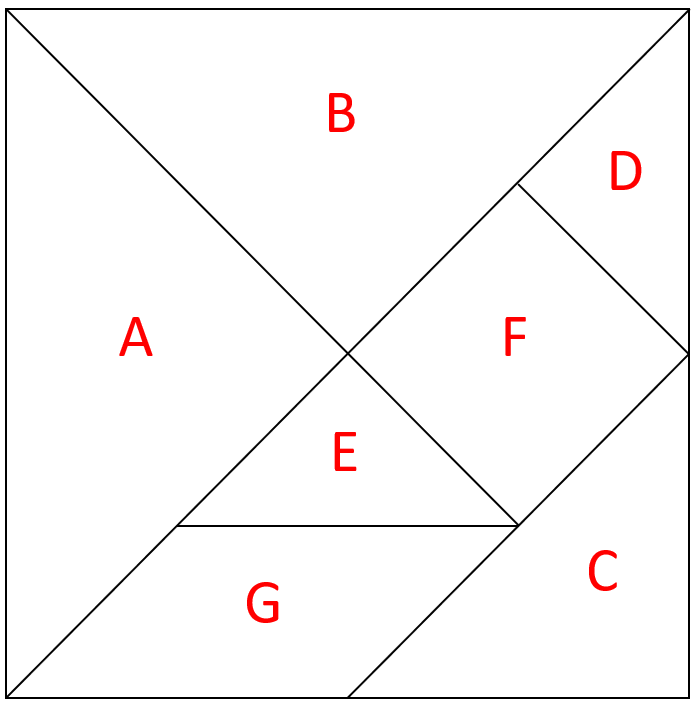Tangram activities (cont.)

Using the pieces created in the last session, create the following shapes and then draw them:

• DEF triangle
• DEF trapezium
• CDEG rectangle
• DEF parallelogram
• DEG rectangle
• CDEG parallelogram
• DEF rectangle
• DEC trapezium
• CDEG trapezium

Origami

Perimeter

## Week 4

Area - Rope activity

• Using a long rope joined together at both ends, find a large space such as outside.
• Step inside the rope with the children so that the rope is behind your waist and everyone is facing in.
• Try making different shapes and ask the children to estimate if the area of each shape is the same or different.
• Return to the classroom and examine the following three shapes which all have the same perimeter of 12.
• The teaching point is that area is affected by shape, not just perimeter.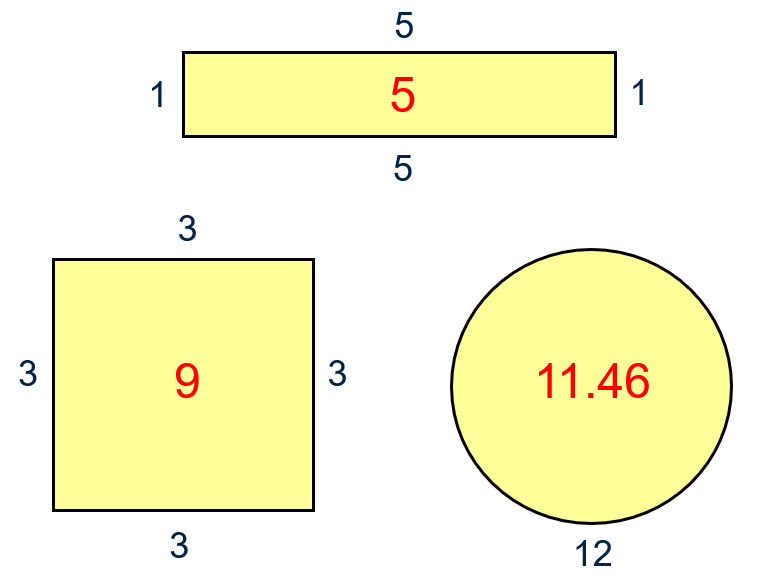Volume

## Week 6

Capacity

Cylinders

Task: Give each child a piece of A4 paper and ask them to make a cylinder.

### Q: Which configuration will produce the greatest volume?

An A4 piece of paper is 210 x 297 mm.  Rounding this off to centimetres would give us 21 x 30 cm.  The following formula is not formally introduced until Years 7 and 8 but the teaching points are:

1. Shape is a determining factor when dealing with volume and area.
2. Mathematical formulas embody these relationships.  Knowing that there is a formula is more important in Year 1 than knowing how to use the formula.
3. Mathematics is not just about questions but can be a way of explaining things.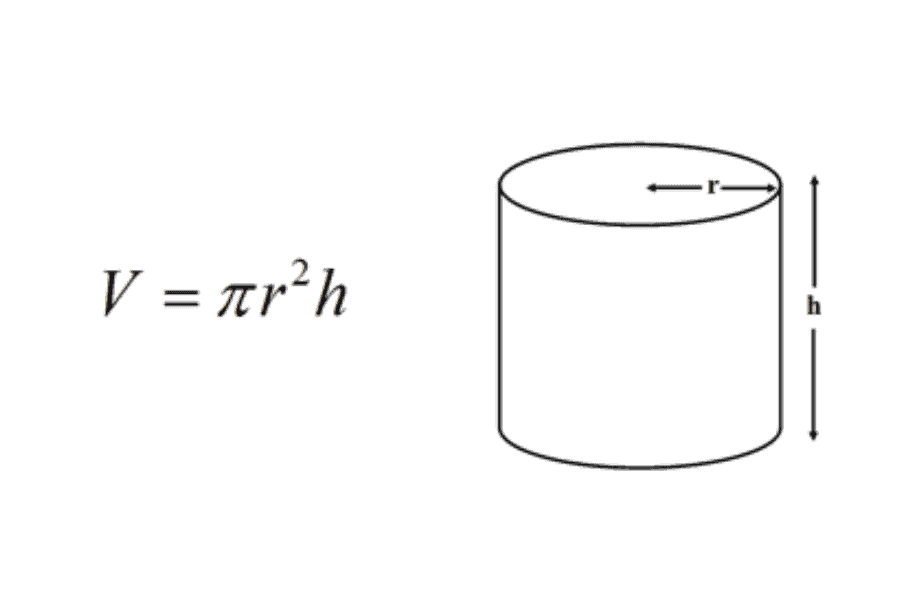(https://www.mometrix.com/blog/what-is-the-formula-for-the-volume-of-a-cylinder/)

There is a handy online calculator available at (https://www.omnicalculator.com/math/cylinder-volume).

## Week 7

Topography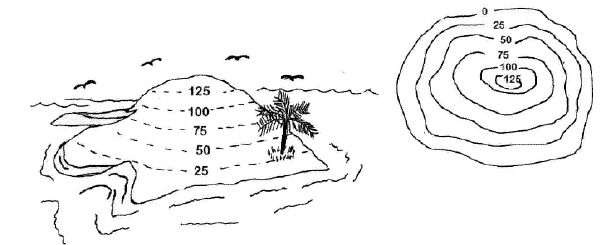https://www.msnucleus.org/membership/html/k-6/uc/geography/5/ucg5_1a.html

## Week 8

Naming shapes and objects

## Week 9

Introduction to Venn diagrams

Backyard analogy of boundaries.

Problem solving with perimeter and area

Problem solving with volume and capacity

## Week 10

Moderated self-assessment